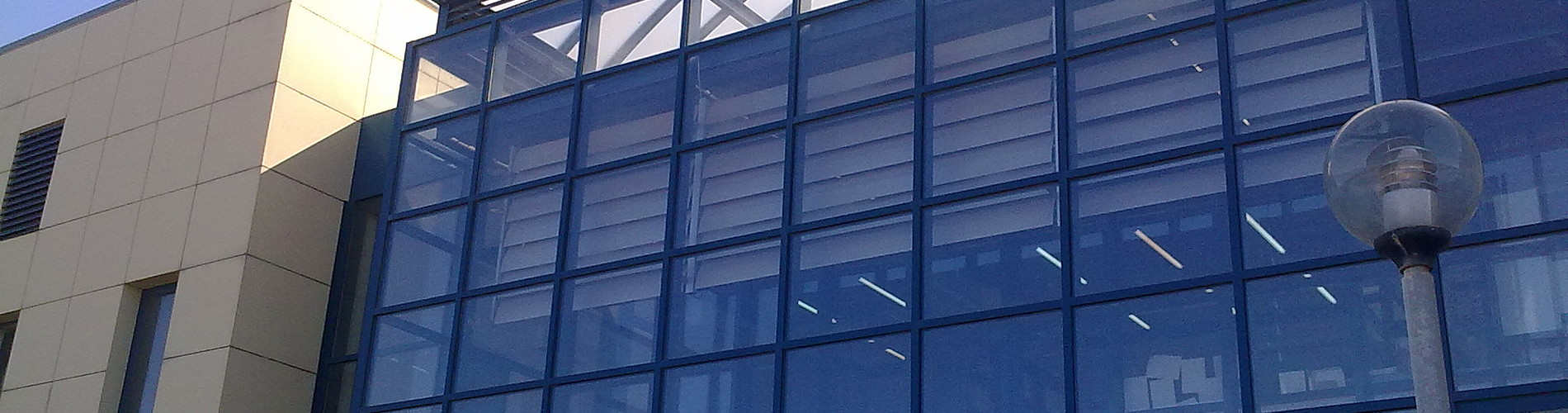School of Chemical and Environmental Engineering

Now offering two distinct diplomas: Chemical Engineering and Environmental Engineering

# Differential and Integral Calculus II

1. COURSE INFORMATION:

 School Environmental Engineering Course Level Undergraduate Course ID MATH 102 Semester 2nd Course Category Required Course Modules Instruction Hours per Week ECTS Lectures and Tutorial Exercises 4 Th=3, E=1, L=0 5 Course Type General Background Prerequisites Instruction/Exam Language Greek The course is offered to Erasmus students No Course URL https//www.eclass.tuc.gr/courses/MHPER295   (in Greek)

2. LEARNING OUTCOMES

 Learning Outcomes This course is continuation of Math 101 and aims to provides the essential mathematical techniques that an engineer needs in the theoretical part of his training as well as  what he/she  shall need in the computational part of his work.On completion of this course students will be expected tounderstand the basic vector operations, addition multiplication of vectors, find the projection of a vector on another vectorfind areas of parallelograms and  volume of parallelepipeds  produced by vectors.find the equations of lines and planes in spacecalculate the partial derivatives of a function of 2or 3 variablescalculate the partial derivatives of functions given in implicit formfind gradient vector and the directional derivative of functions of two or three  variablesfind whether a vector field is the gradient of a real valued functionfind the  extreme values of functions of two variables  using the criterion of the second partial derivativefind the extreme values of functions under constrains using the Lagrange methodevaluate the volumes and areas of solids with the useage of double and triple integralsfind the work of force in a curve and examine whether the force is conservativefind the flow of a fluid  along or across a plane curveevaluate the divergence and rotation of a vector field and their relation with the flow  of fluid  across  along or across a plane curve  (Green’s theorem), the boundary of a surface (Stoke’s theorem) or along a closed surface (Gauss theorem) General Competencies/Skills Review, analyse and synthesise data and information.Work autonomously.Work in teams.Work in an international frame.

3. COURSE SYLLABUS

 Vectors in Two and three Dimensional space. Inner, Cross, triple product. Lines and planes in Space. Polar, Cylindrical and Spherical Coordinates. Curves in the space and their Tangents. Integrals and derivatives of Vector Functions. Velocity, Tangential and Normal components of Acceleration. Functions of two and more variables. Partial Derivatives of functions of several variables, div, grad, Curl. The Chain rule. Directional Derivatives and Gradient vectors. Tangent Planes and differentials. Extreme values and Saddle points. Lagrange multipliers. Line Integrals. Path independence, Conservative Fields. Double and Triple integrals. Applications in Physics and Geometry :Moments and Centers of Mass, Volumes of Solids. Green’s theorem in the plane. Surface Integrals. Stoke’s Theorem. The divergence (Gauss’s) Theorem.

4. INSTRUCTION and LEARNING METHODS - ASSESSMENT

 Lecture Method Direct (face to face) Use of Information and Communication Technology E-class support Instruction Organisation Activity Workload per Semester (hours) - Lectures 39 - Tutorial Exercises 13 - Autonomous study 73 Course Total 125 Assessment Method Ι. Written final examination (100%)